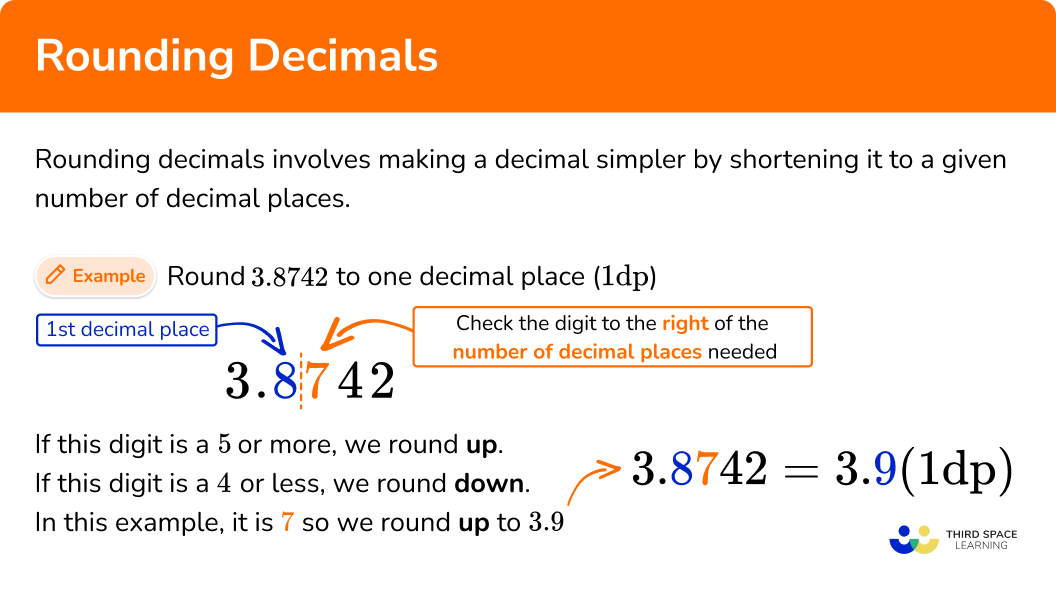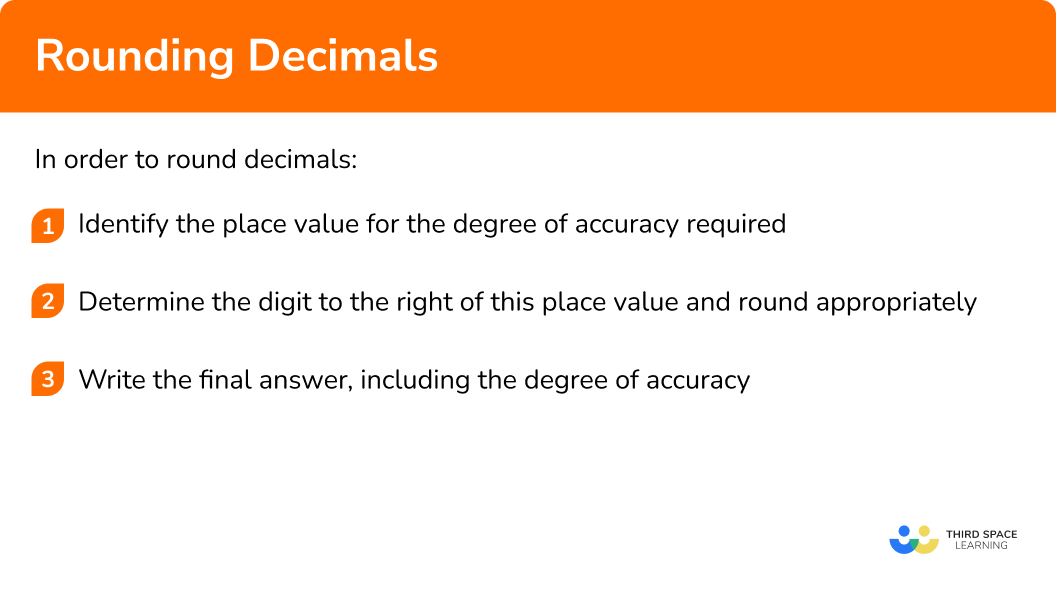GCSE Maths Number Rounding Numbers

Rounding Decimals

# Rounding Decimals

Here we will learn about rounding decimals, including how to round to one, two and three decimal places.

There are also rounding decimals worksheets based on Edexcel, AQA and OCR exam questions, along with further guidance on where to go next if you’re still stuck.

## What is rounding decimals?

Rounding decimals involves making a decimal simpler by shortening it to a given number of decimal places.

To do this we consider the digit to the right of the number of decimal places required and round it as follows,

• If the digit is a \bf{5} or more, we round up.
• If the digit is a \bf{4} or less, we round down.

Let’s look at rounding 3.8742 to one decimal place. This means we need one decimal place only in our answer, so our answer will be either rounded down to 3.8 or rounded up to 3.9. We use rounding rules to help us to decide whether 3.8742 is closer to 3.8 or 3.9.

In this case, we look at the 7 to help us to decide.

7 is more than \bf{5}, so we round up to 3.9.

Now let’s look at rounding 3.8742 to two decimal places.

We need two decimal places in our answer, so our answer will either be rounded down to 3.87 or rounded up to 3.88 .

In this case, we look at the 4 to help us to decide.

4 is less than \bf{5}, so we round down to 3.87.

By rounding a number, we lose the accuracy of the number. Rounding can therefore be used to estimate calculations where rounding to the nearest whole number can make mental calculations easier to compute.

Step-by-step guide: Estimation

### What is rounding decimals?## How to round decimals

In order to round decimals:

1. Identify the place value for the degree of accuracy required.
2. Determine the digit to the right of this place value and round appropriately.
3. Write the final answer, including the degree of accuracy.

### Explain how to round decimals## Rounding decimals examples

### Example 1: round to 1 decimal place

Round 9.37 to 1 decimal place.

1. Identify the place value for the degree of accuracy required.

Here we need to round to 1 decimal place.

2Determine the digit to the right of this place value and round appropriately.

The number to the right of the 1 st decimal place is 7.

7 is greater than 5 so we need to round up by changing the 3 to a 4 and discarding any subsequent values.

3Write the final answer, including the degree of accuracy.

9.37 = 9.4 to 1 decimal place.

We can also write this as 9.4 \ (1dp).

### Example 2: round to 1 decimal place (nearest tenth)

Round 14.4293 to the nearest tenth.

Identify the place value for the degree of accuracy required.

Determine the digit to the right of this place value and round appropriately.

Write the final answer, including the degree of accuracy.

### Example 3: round to 2 decimal places

Round 13.612 to 2 decimal places.

Identify the place value for the degree of accuracy required.

Determine the digit to the right of this place value and round appropriately.

Write the final answer, including the degree of accuracy.

### Example 4: round to 2 decimal places

Round 84.59821 to the nearest hundredth.

Identify the place value for the degree of accuracy required.

Determine the digit to the right of this place value and round appropriately.

Write the final answer, including the degree of accuracy.

### Example 5: round to 3 decimal places

Round 23.5325 to 3 decimal places.

Identify the place value for the degree of accuracy required.

Determine the digit to the right of this place value and round appropriately.

Write the final answer, including the degree of accuracy.

### Example 6: round to 3 decimal places

Round 132.6794215 to the nearest thousandth.

Identify the place value for the degree of accuracy required.

Determine the digit to the right of this place value and round appropriately.

Write the final answer, including the degree of accuracy.

### Common misconceptions

• Decreasing the value of the decimal place when rounding down

When rounding down, the value of the decimal place stays the same, it doesn’t decrease. For example, when rounding 4.62 to one decimal place, the 2 indicates that we need to round down. The correct answer is 4.6 not 4.5.

• Leaving out zeros

When a 9 is increased to 10 and the one rolls over, it is important to include the zeros so that you are giving the answer to the correct number of decimal places. For example, 4.597 to two decimal places is 4.60 rather than 4.6.

• Changing extra digits

It is only the last digit that should be changed when rounding up. For example, 4.318 to two decimal places is 4.32, not 4.42.

Once we have decided whether to round up or down we can discard all subsequent values. For example, 7.693012 to 1 decimal place is 7.7, not 7.793012 or 7.700000.

Rounding decimals is part of our series of lessons to support revision on rounding numbers, and upper and lower bounds. You may find it helpful to start with the main rounding numbers lesson for a summary of what to expect, or use the step by step guides below for further detail on individual topics. Other lessons in this series include:

### Practice rounding decimals questions

1. Round 8.49 to 1 decimal place.

8.48.58.509.5We need to round to 1 decimal place. The digit to the right is 9 so we round up by increasing the 4 to a 5 and discarding subsequent values.

8.49 = 8.5 to 1 decimal place.

2. Round 15.74103 to the nearest tenth.

15.715.7415.7000015.8The tenths digit is the first digit to the right of the decimal point so we need to round to 1 decimal place. The next digit to the right is 1 so we round down by leaving the 7 the same and discarding subsequent values.

15.74103 = 15.7 to the nearest tenth.

3. Round 7.579 to 2 decimal places.

7.577.687.587.60We need to round to 2 decimal places. The digit to the right is 9 so we need to round up by increasing the 7 to an 8 and discarding subsequent values.

7.579 = 7.58 \ (2dp)

4. Round 32.82049 to the nearest hundredth.

32.8232.8332.8200032.81The hundredths digit is the second digit after the decimal point so we need to round to 2 decimal places. The digit to the right is 0 so we need to round down by leaving the 2 the same and discarding subsequent values.

32.82049 = 32.82 to the nearest hundredth.

5. Round 0.0641 to 3 decimal places.

0.0650.0630.06410.064The thousandths digit is the third digit after the decimal point so we need to round to 3 decimal places. The digit to the right is 1 so we need to round down by leaving the 4 the same and discarding subsequent values.

0.0641 = 0.064 \ (3dp)

6. Round 14.7498123 to the nearest thousandth.

14.75014.74914.7514.800We need to round to 3 decimal places. The digit to the right is an 8 which means we need to round up by increasing the 9 to a 10, carrying the 1, and discarding subsequent values.

14.7498123 = 14.750 to the nearest thousandth.

### Rounding decimals GCSE questions

1. Round the number 132.4672 to one decimal place.

(1 mark)

132.5

(1)

2. (a) Calculate \sqrt{47.5}. Write down all the digits on your calculator display.

(2 marks)

(a) 6.892024376

(1)

(b) 6.89

(1)

3. Use the formula C=\pi{d} to find the circumference C of a circle with a diameter d = 10 \ cm.

(3 marks)

C=\pi\times{10}

(1)

=31.41592654

(1)

=31.42 \ cm \ (2dp)

(1)

## Learning checklist

You have now learned how to:

• Round numbers and measures to an appropriate degree of accuracy, for example, to a number of decimal places

## Still stuck?

Prepare your KS4 students for maths GCSEs success with Third Space Learning. Weekly online one to one GCSE maths revision lessons delivered by expert maths tutors.

Find out more about our GCSE maths tuition programme.